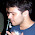## Friday, June 24, 2011

### diagonals and interior points for rectangles

You are given a R rectangle  a x b of integer size. Each rectangle contains unit blocks, consider the diagonal and count how many blocks c are touched by the diagonal. Given a value K how many rectangles have exactly K block touching the diagonal?  here an example

I found this problem online but was not able to solve it so far

1.Since I've a CG background, my answer is: use Bresenham's algorithm

2.gcd(a, b) + a + b - 1

3.x = gcd(a, b)
answer = x + a/x + b/x - 1

4.thanks Stefano, that was good.